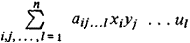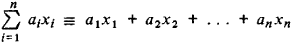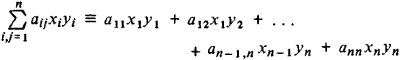# Multilinear Form

(redirected from Alternating multilinear form)

## multilinear form

[′məl·tə‚lin·ē·ər ′fȯrm]
(mathematics)
A multilinear form of degree n is a polynomial expression which is linear in each of n variables.
McGraw-Hill Dictionary of Scientific & Technical Terms, 6E, Copyright © 2003 by The McGraw-Hill Companies, Inc.
The following article is from The Great Soviet Encyclopedia (1979). It might be outdated or ideologically biased.

## Multilinear Form

an algebraic expression of the formThis expression is a polynomial containing m sets of variables, with n variables in each set:

x1, x2, …, xn; y1, y2, …, yn; …; u1, u2, …, un

Each term of the polynomial is of the first degree in a variable from each set. A multilinear form is thus a linear polynomial expression in the variables of one set—hence its name. Special types of multilinear forms include the linear form (m = 1)the bilinear form (m = 2)and the trilinear form (m = 3).